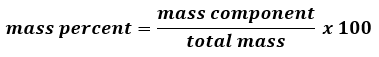# Problem: A binary compound between an unknown element E and hydrogen contains 91.27% E and 8.73% H by mass. If the formula of the compound is E3H8. Calculate the atomic mass of E.

###### FREE Expert Solution

Since the given percentages are % by mass, let’s assume a 100 g of sample of E3H8.Assume Mass of each component (% to g):

87% (65 ratings)###### Problem Details

A binary compound between an unknown element E and hydrogen contains 91.27% E and 8.73% H by mass. If the formula of the compound is E3H8. Calculate the atomic mass of E.